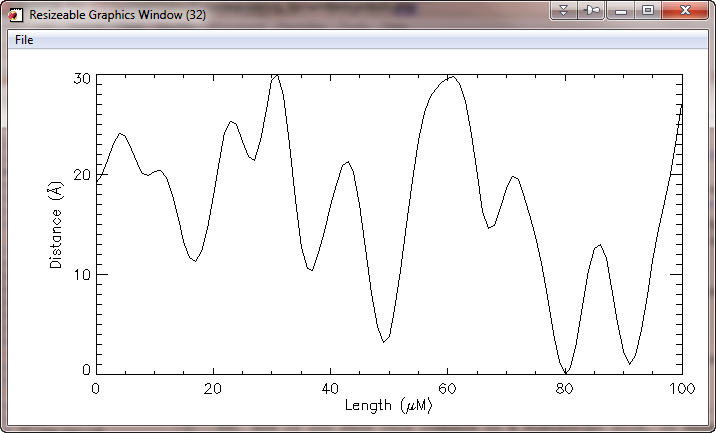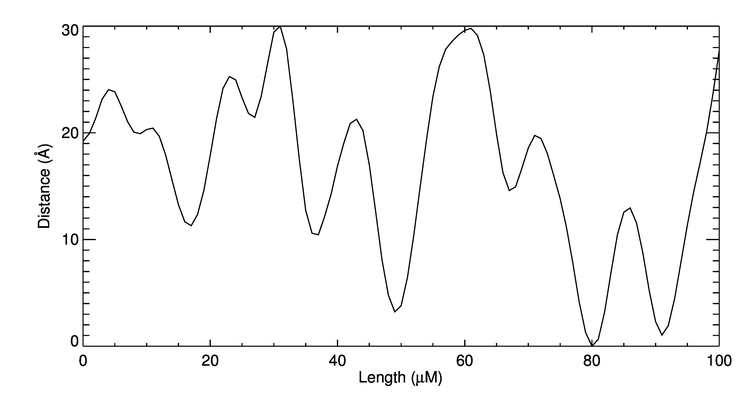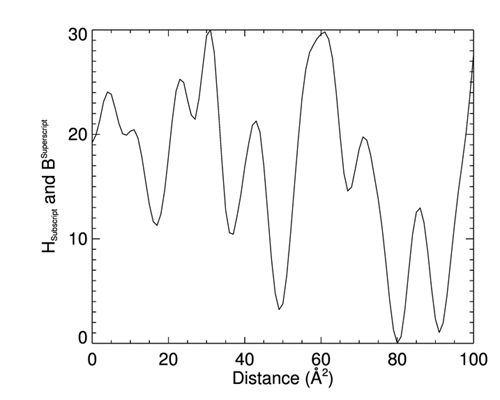# Embedding Greek and Other Symbols in TextQUESTION: I'm looking for a simple, easy way to specify Greek characters, subscripts, superscripts, and other symbols (e.g., a less-than-or-equal sign) in plot titles and other annotations. Do you have anything like that in Coyote Graphics?ANSWER: Yes, as of November 2012, you can embed any Greek character or other symbol supported by cgSymbol into a string that is used for plot or axis titles. This includes superscripts (\up and \exp, which raise the text to superscript level and reduce the size of the text by 62 and 44 percent, respectively) and subscripts (\down and \sub, which act similarly for subscripts). The symbol name is indicated by the characters “$\” before the symbol name, and the character “$” after the symbol name. The Coyote Graphics routine cgCheckForSymbols is used to parse the string and substitute the correct symbol code for the embedded symbol characters.

Here, for example, is how you could use the Greek character mu in the X title of a plot, and the Angstrom symbol squared in the Y title of a plot.

   cgPlot, cgDemoData(1), XTitle='Length ($\mu$M)', YTitle='Distance ($\Angstrom$$\up2)'  The embedded symbols will be evaluated at run-time, so they will be correct both on the display and if you send this command to a PostScript file, or make a high-quality raster file with ImageMagick. Here, for example, is how the figure below was created.  cgPlot, cgDemoData(1), XTitle='Length (\muM)', YTitle='Distance (\Angstrom$$\up2$)', $Output='embedsymbols_1.png', Aspect = 0.66Embedded symbols are rendered correctly on the display and in PostScript and in high-quality raster files. Embedded symbols are also rendered correctly in cgWindow. Here is the same command rendered in cgWindow. The figure below shows the the cgWindow itself, and the high quality PNG file created from it with the cgControl command.  cgPlot, cgDemoData(1), XTitle='Length ($\mu$M)', YTitle='Distance ($\Angstrom$)',$
WXSize=700, WYSize=300, /Window
cgControl, Output='embedsymbols_3.png'Embedded symbols are rendered correctly in cgWindow and its output.## Embedded Superscripts and Subscripts

There is no particular limit to how many characters can be included in superscripts and subscripts. The following embedded symbols can be used.

• $\upTEXT$ — Raise the text in TEXT to superscript level and reduce character size by 62%.
• $\extTEXT$ — Raise the text in TEXT to superscript level and reduce character size by 44%.
• $\downTEXT$ — Lower the text in TEXT to subscript level and reduce character size by 62%.
• $\subTEXT$ — Lower the text in TEXT to subscript level and reduce character size by 44%.

Here is a contrived example.

   cgPlot, cgDemoData(1), Charsize=2.0, $XTitle='Distance ($\angstrom\up2$)',$
YTitle='H$\subSubscript$ and B$\expSuperscript$'


You see the results here.You can have as many characters in superscripts and subscripts as you need.

If you want to embed a Greek symbol or even something like the Sun symbol in a superscript or subscript, you indicate this by prepending the character with "\\" inside the normal superscript or subscript notation. Here, for example, is how you might use the Sun symbol as a subscript.

   cgSet_TTFont, 'dejavusans'
cgPlot, cgDemoData(1), XTitle='R$\sub\\sun$', Charsize=2.0, Output='test.png'


You do the same thing with Greek symbols.

   cgPlot, cgDemoData(1), XTitle='R$\sub\\Omega$', Charsize=2.0, Output='test.png'